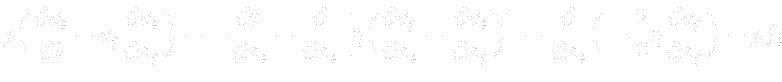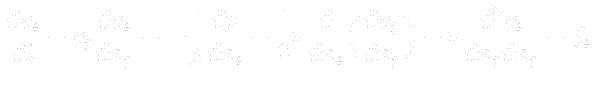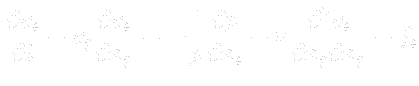Previous: Continuity equation Up: Balance equations in fluid Next: Energy equation
This is an automatically generated documentation by LaTeX2HTML utility. In case of any issue, please, contact us at info@cfdsupport.com.

## Momentum equation

Momentumis vector, thus its conversation has to be made in each direction of all axes. This is its differential form:Whereare volume forces21.4. For constant viscosityequations can be rewritten:For the incompressible fluid () holds and we obtain: